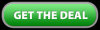Cart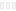Call us to order a book!

#####1-800-551-4754

Monday-Friday 8:00am - 4:30pm CST

## Basic Engine Math and Science to Design High-Performance Engines

You can’t do engine math without numbers. The numbers we use are measured with precision tools or, in some cases, they are assigned values for the purpose of brainstorming proposed modifications and theoretical results. Measurements are taken in what we all recognize as U.S. Customary units of measure such as feet, inches, pounds, and gallons; international SI or metric units such as millimeters, centimeters, and liters are also relevant because many new cars use a combination of both. Once dimensions and other measurements are recorded they can be mathematically manipulated to tell us almost anything we want to know about our engines, including how they might perform and how we might modify them.

Engine math deals with rod and stroke lengths, bearing diameters and clearances, cylinder volumes, bore/stroke ratios, piston weights and speeds, cylinder pressures, atmospheric temperature, and so on.

• Volumes are calculated in cubic inches, cubic centimeters, or liters (appropriate conversion factors are available).
• Engine, piston, and bearing speeds are a function of time so they are measured in feet per minute (ft/min) or feet per second (ft/sec).
• Atmospheric temperature measurements are noted in degrees Fahrenheit.
• Pressure measurements are given in pounds per square inch (psi).

Core Principles

You are probably familiar with the core principles taught in high school science classes. Not surprisingly, all of them apply to engine projects in one way or another. Basic physics affects every function inside your engine and because most of the principles are straightforward and easy to visualize, it’s easy to understand how they affect engine performance.

Before I dive into the heart of the engine to work some basic short-block math, let’s take a brief moment to review some of the core principles that affect engine operation. As you progress through the book, you’ll find that each of the following principles apply to engines in one or more ways.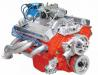The math going on inside your performance engine is easy to grasp once you understand the formulas and how they relate to engine performance.Acceleration

The rate of increase of speed or velocity. In engine math it is usually noted in feet per second (ft/sec) or feet per minute (ft/min). It represents a change in velocity from a state of rest (no motion or speed) to a given speed, or from a given speed to a greater speed over time. Deceleration is a measure of change in velocity from a given speed to a lesser speed or to zero speed.

Area

The extent or measure of a plane surface or the surface of a solid object. Area is represented in square inches or square centimeters. In engine math it might represent the surface of a piston top or the cross-sectional area of an imaginary plane sliced through an intake port at 90 degrees to the direction of flow. It may also represent the open space of a throttle bore through which air passes, commonly called throttle area.

Circumference

The length of a boundary line of a given circle or the perimeter of a closed curve bounding a plane area. Cylinder bores and throttle bores have a fixed circumference which can be converted to an area measurement by squaring the diameter of the given circle and multiplying by the constant 0.7854. Or multiply 2 x pi x the radius of the circle.

Density

The quantity of something by unit of measure or the mass per unit volume of a substance under specified conditions of pressure and temperature. This is a measure of how tightly packed the atoms and molecules are within any given space—weight divided by volume and noted in pounds per cubic inch squared (lbs/in3)2.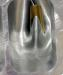All the core elements of math and science are present inside every engine. This view of a supercharged Chevrolet Corvette engine reveals the complexity of component relationships that are governed by basic mathematical principles. (Courtesy Chevrolet Motor Division

Differential

Showing an amount or degree of difference between two quantities such as pressure or temperature. The measurement of difference is the differential and is called the delta (as in delta P for pressure and delta T for temperature). The symbol is the Greek letter D.

Elasticity

The property of certain materials that enables them to return to their original dimensions after an applied stress has been removed. A typical example would be the difference in elasticity (modulus of elasticity) between steel and aluminum connecting rods. Aluminum stretches more than steel so you have to run more pistonto- cylinder-head clearance (than steel) to prevent component contact when the rod material stretches at TDC.

Equation

A mathematical statement asserting that two expressions are equal. It allows you to solve for unknown values by manipulating constants, known values, and variables according to mathematical laws to keep the equation balanced.

Exponent

A number or symbol placed to the right of and above another number, symbol, or expression to indicate the power to which it is raised. The number being raised is called the base number and the exponent indicates the number of times the base number is multiplied by itself. Hence 22 means 2 x 2 = 4 and 33 means 3 x 3 x 3 = 27. In engine math, numbers are typically squared (base number times itself once) or cubed (base number times itself twice).

Force

A vector quantity that tends to produce an acceleration of a body in the direction of its application. Example: In a cylinder, the combustion pressure (force) is constrained in all directions except one and that is the downward motion of the piston when combustion pressure (force) is applied.

Friction

The force that resists the motion of one surface relative to another with which it is in contact.

Fulcrum

The point of support upon which a lever pivots.

Inertia

The unique property of matter that causes it to resist any change in its motion or lack of motion (state of rest). Thus a body at rest remains at rest unless acted upon by an external force and a body in motion continues to move in a linear direction at constant velocity unless acted upon by an external force. Newton’s first law of motion states that the mass of a body is a measure of its inertia.

Lever

The simplest mechanical device, consisting of a rigid bar pivoted about a fixed point and used to transmit a force. Leverage is the action or mechanical advantage of a lever and is represented by the amount of force applied over a given distance from the fulcrum to a load at a given distance on the opposite side of the fulcrum (or somewhere between the fulcrum and the point where force is applied).

Linear

Having only one dimension or direction. Motion A change in the position of a body or component with respect to time as measured by a particular observer in a particular frame of reference.

Percentage

A portion or share in relation to the whole where the whole is represented by 100. It is represented as a fraction or a ratio with 100 as the denominator. Example: 70/100 = 0.70 or 70 percent.

Pressure

The force acting normally on a unit area of surface, or the ratio of force to area. Example: Atmospheric pressure is 14.7 pounds per square inch (14.7 lbs/in2) Absolute pressure (psia) is pressure measured on a gauge that reads zero at zero pressure rather than atmospheric pressure. Gauge pressure (psig) is measured on a gauge that reads zero at atmospheric pressure.

Ratio

A mathematical relationship in degrees or number between two things or quantities. In mathematics it is represented as a fraction. Example: In engine math the simplest ratio is that of crankshaft speed to camshaft speed. If the crankshaft turns at twice the speed of the camshaft, the ratio is 2:1.

Roots

Square roots (√x) and cube roots (3√x) are not often required, but it is good to understand that they are the reverse of exponents. They are tedious to calculate by hand. Fortunately you can find a square root or a cube root quickly on a scientific calculator by pressing the appropriate keys. If a number is squared 32 for example, the square root is expressed as √9 or 3. If a number is cubed (43), the cube root is expressed as 3√64, or 4.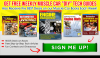Specific Gravity

The ratio of the mass of a given solid or liquid to the mass of an equal volume of distilled water at 4 degrees C (39 degrees F) or of a gas to an equal volume of air or hydrogen under prescribed conditions of pressure and temperature.

Stoichiometric Ratio

The quantitive relationship between reactants and products in a chemical reaction. More specifically, in engine math the stoichiometric ratio of 14.7:1 represents the ideal air-fuel ratio for combustion (for gasoline) that creates minimal by-products.

Vector

A quantity such as velocity completely specified by magnitude (strength) and direction.

Volume

The amount of space occupied by a three-dimensional object or a region of space.

Variable

A quantity capable of assuming any assigned value or a symbol representing such a quantity.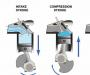The four cycles of operation illustrate the relatively low pressures on the inlet and compression strokes versus the high pressures occurring on the power and exhaust strokes. Mathematical equations are used to describe and calculate the dynamic relationships that occur within the cylinders during each of these cycles.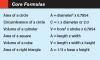If you had no problem grasping these basic concepts, you’re ready to tackle any of the engine math calculations found in this book. Keep in mind that your calculations are only as accurate as your measurements. They say that numbers never lie and that is almost true. If you provide inaccurate measurements, the numbers won’t lie, but they won’t give the right answer either. Now grab your calculator and dive into the easy engine math formulas. They’re going to be more useful and more fun than you expect.

Written by John Baechtel and Posted with Permission of CarTechBooks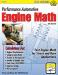GET A DEAL ON THIS BOOK!

If you liked this article you will LOVE the full book. Click the button below and we will send you an exclusive deal on this book.